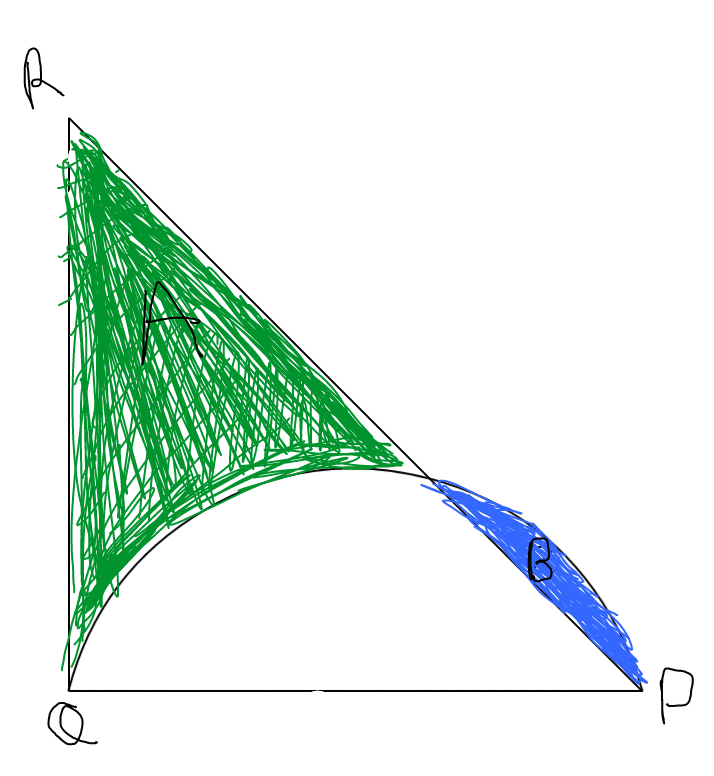# NMTC 2014(Junior level)

Geometry Level 3In the given figure, $PQ=42\text{ cm}$. $QR$ is the tangent to the semicircle at $Q$. If the difference of the areas of region $A$ and $B$ is $357\text{ cm}^2$, then the base $QR$ of the right triangle $PQR$ is (in cm)(Take $\pi=\frac{22}{7}$)

Note: This problem has appeared in nmtc 2014 junior level.

×

Problem Loading...

Note Loading...

Set Loading...